# Python Program to Search an Element in a List

This article deals with some programs in Python that searches an element in a list. Here both element and list must be entered by user at run-time. Here are the list of programs covered in this article:

• Search an element in a list of 5 elements
• Search an element in a list of n elements
• Check if a value exists in a given list, using in operator

## Search an Element in a List

The question is, write a Python program to search a given element from a given list. The program given below is answer to this question:

```mylist = []
print("Enter 5 elements for the list: ")
for i in range(5):
val = int(input())
mylist.append(val)

print("Enter an element to be search: ")
elem = int(input())

for i in range(5):
if elem == mylist[i]:
print("\nElement found at Index:", i)
print("Element found at Position:", i+1)```

Here is its sample run: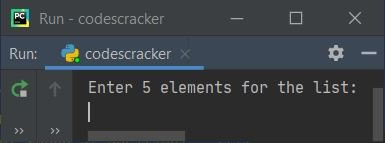Now supply the input say 10, 20, 30, 40, 50 as five elements and 10 as element to be search, then press `ENTER` key to search and print the index and position of the given element in the list like shown in the sample output given below: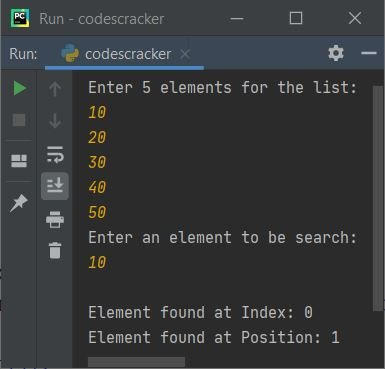## Search an Element in a List of n Elements

After modifying previous program, I've created another program, that is the program given below. This program allows user to define the size of list along with its element and the element that is going to be search from the given list. Let's take a look at the program and its sample run given below:

```mylist = list()

print("Enter the size of list: ", end="")
tot = int(input())

print("Enter", tot, "elements for the list: ", end="")
for i in range(tot):
mylist.append(input())

print("\nEnter an element to be search: ", end="")
elem = input()

for i in range(tot):
if elem == mylist[i]:
print("\nElement found at Position:", i+1)```

Here is its sample run with user input, 6 as size and 50, 51, 52, 53, 54, 55 as six elements, and 51 as element to be search: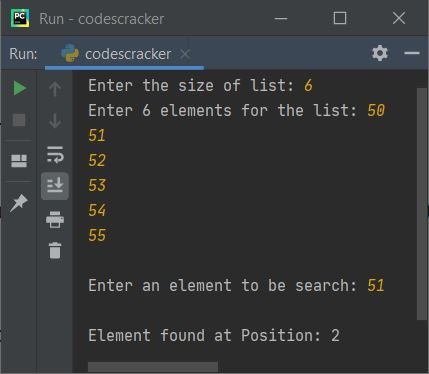Here is another sample run with user input 6 as size, p, y, t, h, o, n as six elements, and p as element to be search: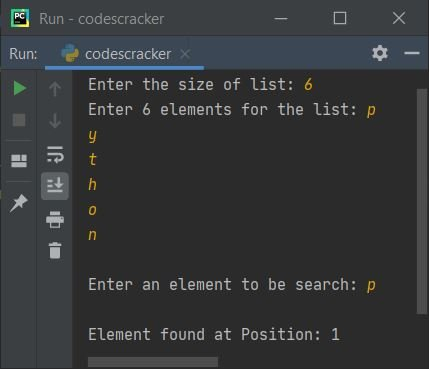#### Modified Version of Previous Program

This program is created to deal with conditions like, when element does not found, only one element found, duplicate element found.

```mylist = list()

print("Enter the size of list: ", end="")
tot = int(input())

print("Enter", tot, "elements for the list: ", end="")
for i in range(tot):
mylist.append(input())

print("\nEnter an element to be search: ", end="")
elem = input()

poslist = list()
for i in range(tot):
if elem == mylist[i]:
poslist.append(i+1)

if len(poslist) == 0:
elif len(poslist) == 1:
print("\nElement found at position:", poslist)
else:
print("\nElement found at positions:", end="")
for i in range(len(poslist)):
print(poslist[i], end=" ")```

Here is its sample run with user input, 6 as size and 1, 2, 3, 2, 4, 2 as six elements, and 2 as element to be search: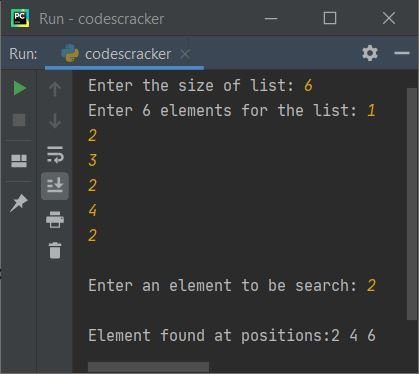Here is another sample run with user input, 4 as size, 10, 20, 40, 50 as four elements, and 30 as element to be search: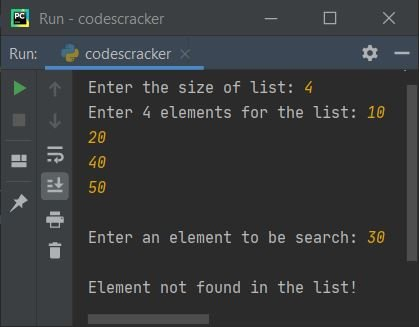## Python Check if Value Exists in List using in Operator

To check whether a particular element or item is available inside the list or not in Python, then use the in operator as shown in the example given below.

```my_list = ["one", "two", "three", "four"]
if "two" in my_list:
print("\n'two' is in the list.")
else:
print("\n'two' is not in the list.")```

Here is the sample output produced by the above example of checking if a value exists in the list or not in Python.

`'two' is in the list.`

Now let's modify the above code with a program that allows user to define the size of the list along with its elements. Also allows to enter an element to check whether it is available in the given list or not, using the in operator of course.

```print("Enter the size of list: ", end="")
tot = int(input())
print("Enter", tot, "elements: ", end="")
x = []
for i in range(tot):
x.append(input())

print("\nThe list is:")
print(x)

print("\nEnter an element to search: ", end="")
element = input()
if element in x:
print("\nIt is available in the list")
else:
print("\nIt is not available in the list")```

The snapshot given below shows the sample run with user input 4 as size, codes, cracker, dot, com as four elements, and dot as element to check for its availability in the list:Python Online Test

« Previous Program Next Program »